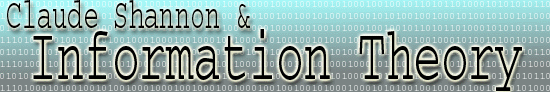Bits and Binary Digits A binary digit is a 1 or a 0. In binary, or base 2, there are only two digits whereas in decimal, or base 10, there are ten digits. Claude Shannon's information theorems work most naturally in binary, and it is mainly because of him that the digital standard has been adopted for sending information, earning him the title "The Father of the Digital Age." A bit in information theory is not a binary digit, although the two are often confused. Understanding the definition of "bit" requires an understanding of Shannon's formula for information, h(p) = -logb(p). The base b can be assigned so one unit of information represents a choice between two equally likely possibilities. Assume that there are two symbols, S1 and S2 each with a 50% probability of being chosen.. Then in the above formula, p = .5 and h = -logb(.5) = logb(2) = 1 under the assumptions. This implies b = 2. Because we have chosen base 2 for the information formula, one unit of information is called a bit. These two definitions are not independant, however. If we assign the binary digit 1 to S1 and 0 to S2, then the single binary digit code we have chosen holds one bit of information, representing the choice between two equally likely possibilities. Formally, this can be expressed as h(.5) = -log2(.5) = 1 for S1 and similarly for S2. If, on the other hand, the probability of S1 is .75 and the probability of S2 is .25, then the number of bits for the symbol S1 is -log2(.75) = 0.41 and the number of bits for S2 is -log2(.25) = 2.0. Intuitively, this makes sense, because S1 would be expected with greater certainty if it has a higher probability, and therefore its appearance would yield less information. Another example of coding where each binary digit yields one bit of information occurs in Fano codes. Actually, the above example was a very simple Fano code.